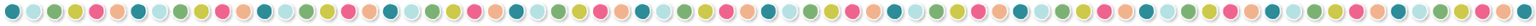# More Thoughts on Teaching LimitsIn my previous post, 3 Tips Before Teaching Limits, we set the stage for helping our Pre-Calculus students prepare for their journey into the world of Calculus.  You can read those helpful hints HERE. Let’s dig into the methods for teaching and finding limits.

Introduce the definition of a limit and explain that intuitively, when we say “as x approaches c, the limit of f(x) approaches L” we mean that as x gets close to c, then f(x) gets close to a limit of L (in other words, y gets close to a real number).

Be sure to explain the notation for one-sided limits and general limits!

Begin with some graphs that are continuous and some that are not continuous.

When students know their graph is continuous, direct substitution works. That’s easy!  But, when the graph is not continuous at the “target value, c, the value x is approaching,” then the trouble begins!

If substitution leads to 0/0 or ∞/∞ students must decide whether there is a limit or no limit. The RULE OF FOUR is the “best practice” to implement while exploring the various procedures like graphing, tables, factoring, algebraic techniques, special trigonometric rules, and later with L’Hopital’s Rule.

## Graphically and Numerically

Initially, I prefer a discovery approach to promote student development of mathematical language that will lead to a deeper understanding of limits. By presenting both piecewise and rational functions, you can help spur great discussion and cognition.

Consider the four examples below, there is rich discussion available among this set. Algebra can be applied on the first prompt. In the second example, a calculator is helpful, but be sure to introduce direct substitution as a first approach to discuss the indeterminate form of 0/0.  Explore the table feature for very small changes in x. Begin the conversation about what should be done when confronted with division by zero. Then, proceed to the piecewise functions and graphing.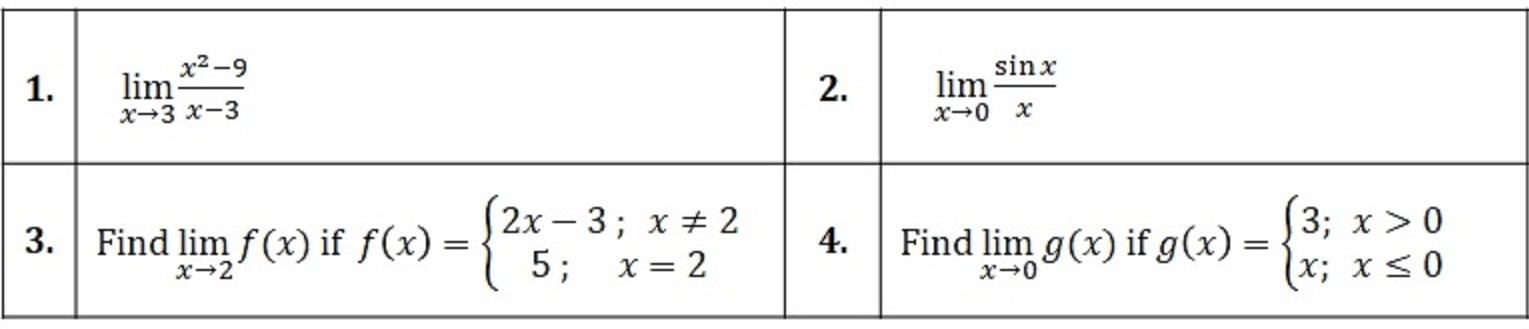Before you move toward algebraic techniques, be sure students understand the notation for one-sided limits versus general limits. For the limit to exist, the limit must be a single value that the function approaches. Here, my favorite is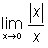.

Finally, be sure to explore a few rational functions using tables to investigate the left and right side of some vertical asymptote. This will set the stage for understanding the vocabulary that “the limit does not exist,” but why the unbounded behavior can lead to a choice of infinity or DNE as a response. Just consider the following three functions as as x approaches zero, to help them discover when to give the appropriate answer: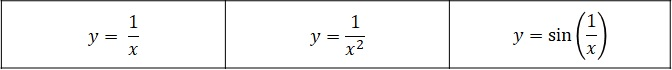In summary, students should notice that the limit of a function DOES NOT EXIST if:

• The same numerical value is not approached from both sides (left and right-hand limits) of the given value.
• The same infinite direction is not approached from both the left- and right-hand side of the given value. (In other words, rational functions have two possible outcomes.)
• The function oscillates so rapidly that a single value is not approached.

At this place, you can introduce Properties of Limits as a sub-topic here. Be sure to download a free infographic on this HOT TOPIC- PROPERTIES OF LIMITS HERE!

## TIP #5: ALGEBRAIC METHODS

Before diving into the algebraic techniques, help students summarize the four possible outcomes when trying direct substitution: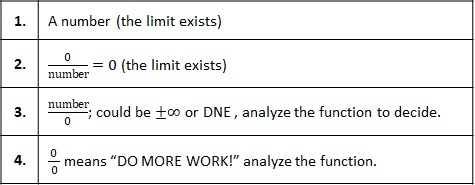Keep reminding students to try direct substitution first, it helps them decide which avenue to take, whether it be a table or graphical approach, factor and simplify, rationalize and simplify, trigonometric properties, or L’Hopital’s Rule, after derivatives are taught.

## TIP #6: LIMITS AND INFINITY

The final exploration of limits will help students understand that the definitions of asymptotes for rational functions are directly related to limits and infinity.

To find vertical asymptotes, look for the roots of the denominator, that is, where the denominator is undefined.  Remember that if 0/0 occurs, there is a hole at that point.  If a (number/0) situation occurs, there is a vertical asymptote. Analyze the one-sided limits to answer DNE or ±∞.

Vertical asymptotes have infinite limits while horizontal asymptotes are defined as limits at the end behavior as they approach a real number.  To find limits at infinity, the “best practice” method is to divide each term in the rational expression by the highest power of x. Then, it’s possible to arrive at an answer by using the rational function graph below and the four following outcomes: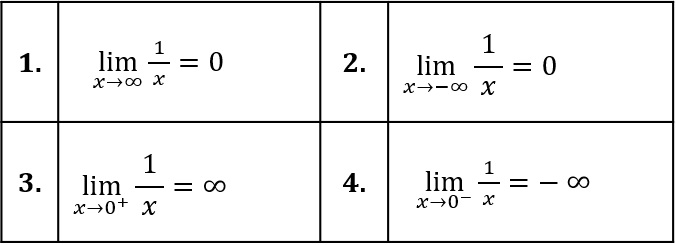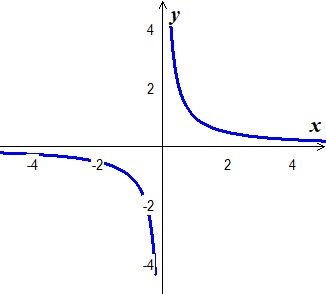In the last post of this three part series on Teaching Limits, we will explore L’Hopital’s Rule.

Stay informed by signing up for our newsletter!## You may also like...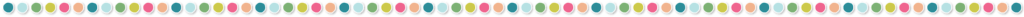This site uses Akismet to reduce spam. Learn how your comment data is processed.

## Let's connect